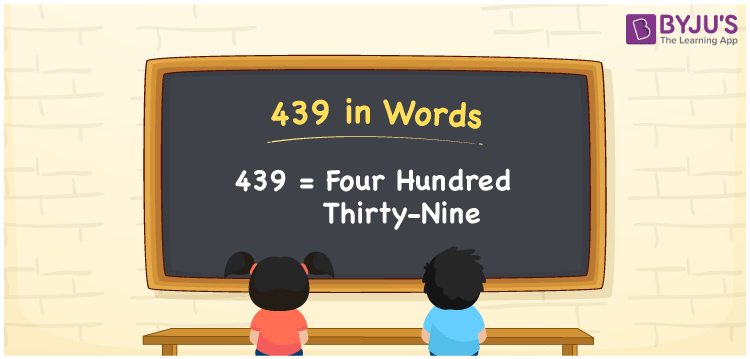# 439 in Words

439 in words is written as Four Hundred Thirty-nine. The name of number 439 in English is “Four Hundred Thirty-nine”. The word Four Hundred Thirty-nine is used in many real-life situations. For example, there are Four Hundred Thirty-nine students in a classroom. Thus, it is a counting number. Also, to represent the currency equal to 439, we can write it in words as Rupees Four Hundred Thirty-nine or Four Hundred Thirty-nine rupees. Therefore, it is necessary to learn the numbers in words, for the ease of understanding and expressing them.

 439 in words Four Hundred Thirty-nine Four Hundred Thirty-nine in Numbers 439

## 439 in English Words## How to Write 439 in Words?

If we know the place value of digits of 439, then we can easily express it in words. The place value is basically the position of a digit in a number. 439 is a three-digit number, therefore, we can specify the position of each digit of 439 in a place value chart. In the Indian numbering system, the order of place value of digits from right to left is given by:

 Hundreds Tens Ones 4 3 9

We can write it in expanded form as:

4 × Hundred + 3 × Ten + 9 × One

= 4 × 100 + 3 × 10 + 9 × 1

= 400 + 30 + 9

= 439

= Four Hundred Thirty-nine

Therefore, 439 in words is written as eight hundred thirties.

439 is a natural number that precedes 440 and succeeds 438.

439 in words – Four Hundred Thirty-nine

Is 439 an odd number? – Yes

Is 439 an even number? – No

Is 439 a perfect square number? – No

Is 439 a perfect cube number? – No

Is 439 a prime number? – Yes

Is 439 a composite number? – No

## Frequently Asked Questions on 439 in Words

Q1

### How to write 439 in words?

439 in English is written as “Four Hundred Thirty-nine”.
Q2

### Is 439 an odd number or even number?

439 is an odd number because it is wholly divisible by 2.
Q3

### Write Four Hundred Thirty-nine in numbers.

Four Hundred Thirty-nine in numbers is 439.